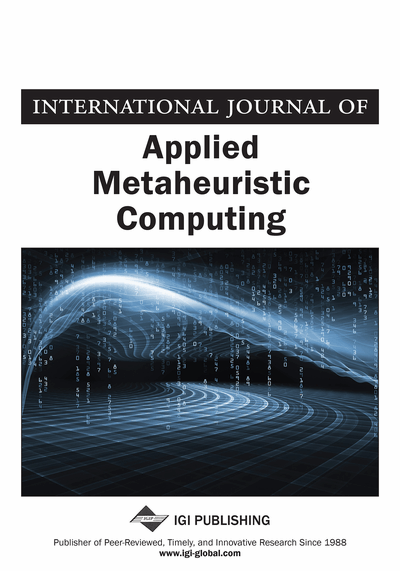# The Strict Strong Coloring Based Graph Distribution Algorithm

Nousseiba Guidoum (MISC Laboratory, University Mentouri Constantine, Constantine, Algeria), Meriem Bensouyad (MISC Laboratory, University Mentouri Constantine, Constantine, Algeria) and Djamel-Eddine Saïdouni (MISC Laboratory, University Mentouri Constantine, Constantine, Algeria)
DOI: 10.4018/jamc.2013010104

## Abstract

State space explosion is a fundamental obstacle in formal verification of concurrent systems. As a solution for this problem, this paper deals with distributed state space. The authors’ solution is to introduce the coloring concept and dominance relation in graphs for finding the good distribution of given graphs. This basic solution is improved in two steps: the initialization and optimization step. The authors also report on a thorough experimental study to evaluate the performance of this new algorithm which depends strongly on the size, nature of the graphs, and the chosen number of workers. In addition, the quality of this algorithm is illustrated by comparison with the hash function (MD5) based algorithm. To the best of the authors’ knowledge, it is the first time when coloring concept is used to solve this problem.
Article Preview
Top

## 1. Introduction

Formal verification is one of the main approaches to aid engineers on the development and validation of concurrent systems. In this approach, system’s behavior can be modeled as a state space. The set of states forms a graph where states are connected if there is an action that can be executed to transform the first state into the second. The graphs, modeling finite state systems, can be explored exhaustively because of the graph size and the exploration time which may grow exponentially with the size of the system description. In this case, the formal verification process becomes more and more slowly and may not be terminated. This problem is known as state space explosion problem (Bérard et al., 2001; Clarke et al., 1999; Valmari, 1998). One solution consists in the distribution of the graph. Note that, the need for parallel and distributed computing becomes inevitable to deal not only with the presented problem but with all applications using large scale graphs. Graph distribution is a well known optimization problem (Bixby et al., 1993). It occurs in applications on many areas, others than formal verification, like parallel computing, communication protocols, distributed algorithms, and industrial case studies.

Several factors should be taken into account to have a good distribution. The most important of them are the workload balancing of the workers (i.e. no unemployed or overloaded workers) and the minimization of the distribution cost (i.e. edges to be cut).

Distributing the state space among several workstations (workers) which communicate through a message in a network is the subject of this paper. A new heuristic based on coloring concept and dominance relation in graphs is presented to find a good distribution within a reasonable time.

Many papers present several approaches for solving this NP-hard problem (Bixby et al., 1993) and other distributed applications on large graphs (Bouneb & Saïdouni, 2009; Orzan et al., 2005; Stanton & Kliot, 2011). Authors in Saad et al. (2009) have presented deeply the different solutions proposed up to 2009. All these solutions are based on a partition function which assigns each state to a fixed worker. These approaches differ mainly by the nature of this function. In Stanton and Kliot (2011), authors have used ten heuristics such that each one of them gives an algorithm for selecting the index of the partition where a state is assigned. However, coloring approach has not yet used for distributing graphs. Graph coloring has been studied profoundly in literature (Dharwadker, 2006; Klotz, 2002). As a variant, graph strict strong coloring has been defined in Haddad and Kheddouci (2009) where an exact algorithm for trees has been proposed. This coloring approach has been extended for general graphs (Bouzenada et al., 2012) which is named GGSSCA (for Generalized Graph Strict Strong Coloring Algorithm).

In this paper, the proposed approach is the first heuristic algorithm, for resolving the state space distribution, based on GGSSCA (Bouzenada et al., 2012). Particularly, we use its first step which insures the dominance property to make the initial distribution. After that, another process will be started to build the final and optimal distribution. The goal of the proposed approach is, given a graph G as input and a number W, to find the better distribution of G which respects the balancing constraint (i.e. holds good load balancing), and minimizes the communication costs (i.e. edges between states on different parts).

## Complete Article List

Search this Journal:
Reset
Open Access Articles: Forthcoming
Volume 12: 4 Issues (2021): Forthcoming, Available for Pre-Order
Volume 11: 4 Issues (2020): 3 Released, 1 Forthcoming
Volume 10: 4 Issues (2019)
Volume 9: 4 Issues (2018)
Volume 8: 4 Issues (2017)
Volume 7: 4 Issues (2016)
Volume 6: 4 Issues (2015)
Volume 5: 4 Issues (2014)
Volume 4: 4 Issues (2013)
Volume 3: 4 Issues (2012)
Volume 2: 4 Issues (2011)
Volume 1: 4 Issues (2010)
View Complete Journal Contents Listing